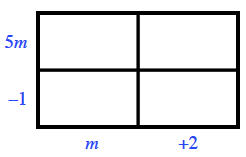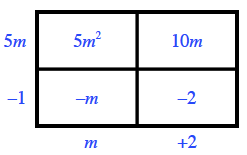Home > CCA > Chapter 11 > Lesson 11.3.5 > Problem11-136

11-136.

Multiply the expressions below using generic rectangles. Homework Help ✎

1. $(5m−1)(m+2)$

Make your generic rectangle and label the dimensions.Find the area of each of the sections, then add the areas together to get your answer.$5m^2+9m−2$

1. $(6−x)(2+x)$

See the help for part (a).

$12+4x−x^2$

1. $(5x−y)^2$

See the help for part (a).

1. $3x(2x−5y+4)$

See the help for part (a).